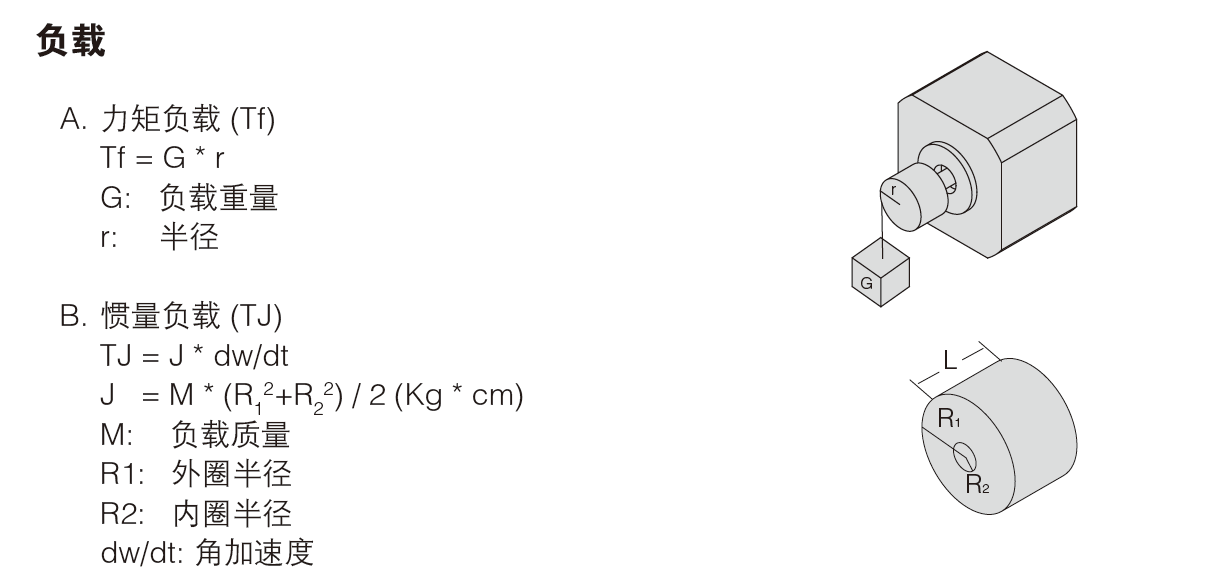1.克服驱动机构的摩擦转矩Tf

2.克服负载和电机转子惯量的启动转矩TjT=2×10-4×157-0/0.1=0.314Nm

f2– f1=1600pps

ta=0.04s

T=2.5×10-4×（π/180）×1.8×1600/0.04=0.314Nm

J1=WD²/8G²=0.01/8×32=0.011kg·cm²

J2=WD²/8G²=0.15×62/8×3/62=0.169kg·cm²

W为重量，D为直径，G为减速比（皮带轮直径/滚轮直径）

J3=W/4D²=0.04/432=0.09kg·cm²

W为重量，D为皮带轮直径。

J4=0.023kg·cm²

J=J1+J2+J3+J4=0.293kg·cm²2）电机的转速:

300×60/30=600rpm

6×600/1.8=2000pps

=0.0213Nm